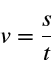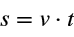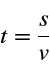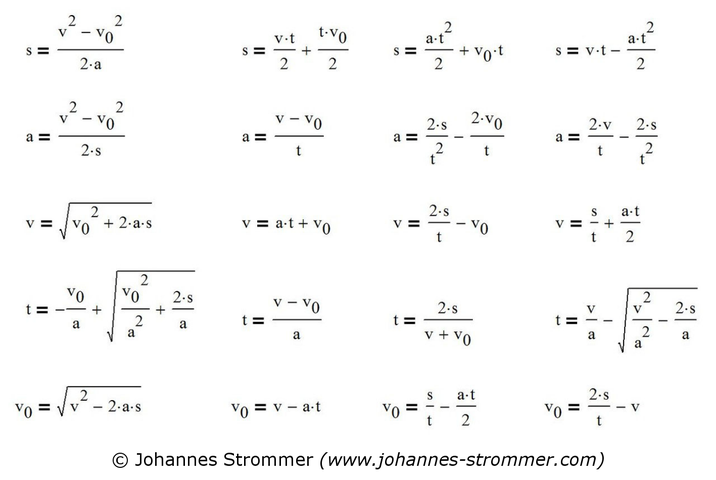# Formulas for Speed, Acceleration, Distance & Time

On this page you will find all formulas for the calculation of speed, acceleration, distance and time with or without initial speed. At the end of the page there is a example for a better understanding of the formulas, in which the acceleration, the final velocity and the average velocity are calculated.

## Formulas for Average Velocity, Distance and Time

The following formulas can be used to calculate the average speed, the distance or the time required. You only need to remember one of these formulas, as the other formulas can be obtained by simply rearranging them. The formula on the far right represents the correct mathematical notation and is also called the difference quotient:### Meaning of the Variables

 v average velocity in m/s in the interval [t0; t1] s bzw. Δs distance or route in m in the interval [t0; t1]; sometimes d is used instead of s t bzw. Δt time required in s s(t1) distance at time t1 s(t0) distance at time t0 (this value is usually 0)

Attention to the units:
The units must always fit together! To convert a speed v given in km/h into m/s, simply divide the speed by 3.6:
v = 18 km/h --> 18/3.6 --> v = 5 m/s

If you multiply a speed v given in m/s by 3.6, you get the same speed in km/h:

v = 10 m/s --> 10⋅3.6 --> v = 36 km/h

Other possibility:
If you take distance in km and time in h, you get speed in km/h.

## Formuals for Uniform Acceleration - Starting Velocity ≠ 0

The following formulas apply to a uniform (= constant) acceleration or deceleration (= braking, negative acceleration) only with an initial velocity not equal to 0. An initial distance is not considered in the formulas, since this is not relevant for most tasks.

A negative value for the acceleration means that the vehicle is actually braking or decelerating.

Three sizes must always be known!Formulas for calculating braking distance, acceleration, (starting) velocity and time while starting velocity is not 0; condition: constant acceleration.

### Meaning of the Variables

 v final velocity in m/s v0 starting velocity in m/s a acceleration or deceleration in m/s² s (braking) distance in m t time needed in s

## Formulas for Uniform Acceleration - without Starting Velocity

These formulas apply to constant acceleration and deceleration, whereby both the initial velocity and the initial distance must be zero.

In principle, these are the same formulas as above, but the initial speed v0 is set to zero. The 5th line in the formula collection above is completely omitted.

Two sizes must always be known!

See the previous chapter for the meaning of the variables.Formulas for an initial velocity equal to 0; condition: constant acceleration.

### Simple Example

This example shows that the above formulas can also be easily used in practice. You only need a stopwatch, which is available on every smartphone, and a measuring tape.

#### Specification

A garden train takes 6 seconds to reach the maximum speed by uniform acceleration, covering a distance of 9 m. Asumed that the initial speed is 0 m/s (acceleration from standstill)

• the acceleration,
• the final velocity and
• the average speed has to be calculated.

#### Calculation of acceleration

If you insert in the formula of the 2nd line last column you get the acceleration you are looking for:
a = 2⋅s/t² = 2⋅9 m/(6 s)² --> a = 0.5 m/s²

#### Calculation of the final velocity

Now you can easily calculate the speed by simply choosing one of the three formulas out of the 3rd line. If you use the 2nd formula you get:
v = a⋅t = 0.5 m/s²⋅6 s --> v = 3 m/s
If you want to know the speed in km/h, multiply v by 3.6: 3⋅3.6 = 10.8 km/h

#### Calculation of the average speed

Inserting in formula v = s/t results in:
v = 9 m / (6 s) --> v = 1.5 m/s = 1.5⋅3.6 km/h = 5.4 km/h

As you can see, the average speed is only half the final velocity.

## Current Velocity & Momentary Acceleration

If the acceleration is not constant, the use of the above formulas is not allowed. Instead, one calculates the acceleration, the velocity or the distance by differential or integral calculation.

### Main Formulas

The current velocity v(t) at any time t is calculated by deriving the function of distance s(t) once from time t (= differential quotient):

If the momentary acceleration is known, the function of acceleration a(t) must be integrated after time t in order to get the current velocity v(t):

You get the momentary acceleration a(t) by deriving the function of speed v(t) once or by deriving the function of distance s(t) twice after time t: